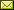## Simple Fixpoint Iteration To Solve Parity Games

 Tom van Dijk (University of Twente) Bob Rubbens (University of Twente)

 A naive way to solve the model-checking problem of the mu-calculus uses fixpoint iteration. Traditionally however mu-calculus model-checking is solved by a reduction in linear time to a parity game, which is then solved using one of the many algorithms for parity games. We now consider a method of solving parity games by means of a naive fixpoint iteration. Several fixpoint algorithms for parity games have been proposed in the literature. In this work, we introduce an algorithm that relies on the notion of a distraction. The idea is that this offers a novel perspective for understanding parity games. We then show that this algorithm is in fact identical to two earlier published fixpoint algorithms for parity games and thus that these earlier algorithms are the same. Furthermore, we modify our algorithm to only partially recompute deeper fixpoints after updating a higher set and show that this modification enables a simple method to obtain winning strategies. We show that the resulting algorithm is simple to implement and offers good performance on practical parity games. We empirically demonstrate this using games derived from model-checking, equivalence checking and reactive synthesis and show that our fixpoint algorithm is the fastest solution for model-checking games.

In Jérôme Leroux and Jean-Francois Raskin: Proceedings Tenth International Symposium on Games, Automata, Logics, and Formal Verification (GandALF 2019), Bordeaux, France, 2-3rd September 2019, Electronic Proceedings in Theoretical Computer Science 305, pp. 123–139.
Published: 18th September 2019.

 ArXived at: https://dx.doi.org/10.4204/EPTCS.305.9 bibtex PDF
References in reconstructed bibtex, XML and HTML format (approximated).
 Comments and questions to:eptcs@eptcs.org For website issues:webmaster@eptcs.org